﻿ Excel常用函数公式20例 - 微式办公网

# Excel常用函数公式20例

1、IF函数条件判断

IF函数是最常用的判断类函数之一，能完成非此即彼的判断。

=IF(B4>=9,"合格","不合格")IF，相当于普通话的“如果”，常规用法是：
IF(判断的条件,符合条件时的结果,不符合条件时的结果)

2、多条件判断

=IF(AND(B2="生产",C2="主操"),"有","无")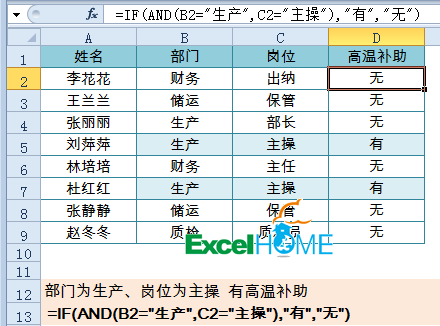AND函数对两个条件判断，如果同时符合，IF函数返回“有”，否则为无。

3、条件求和

=SUMIF(D2:D5,F2,C2:C5)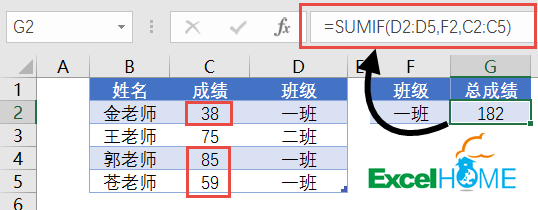SUMIF用法是：
=SUMIF(条件区域,指定的求和条件,求和的区域)

4、多条件求和

=SUMIFS(D2:D9,B2:B9,F2,C2:C9,G2)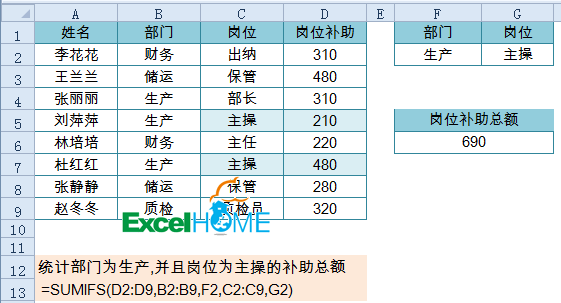SUMIFS用法是：
=SUMIFS(求和的区域，条件区域1,指定的求和条件1,条件区域2,指定的求和条件2,……)

5、条件计数

=COUNTIF(B2:B12,E3)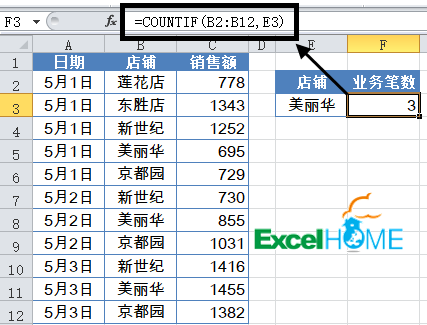COUNTIF函数统计条件区域中，符合指定条件的单元格个数。常规用法为：
=COUNTIF(条件区域,指定条件)

6、多条件计数

=COUNTIFS(B2:B9,F2,C2:C9,G2)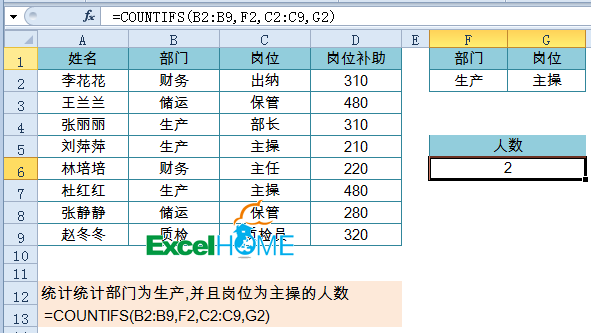COUNTIFS函数统计条件区域中，符合多个指定条件的单元格个数。常规用法为：
=COUNTIFS(条件区域1,指定条件1,条件区域2,指定条件2……)

7、条件查找
VLOOKUP函数一直是大众情人般的存在，函数的语法为：
VLOOKUP(要找谁,在哪儿找,返回第几列的内容,精确找还是近似找)

=VLOOKUP(\$F\$5,\$B\$1:\$D\$10,2,0)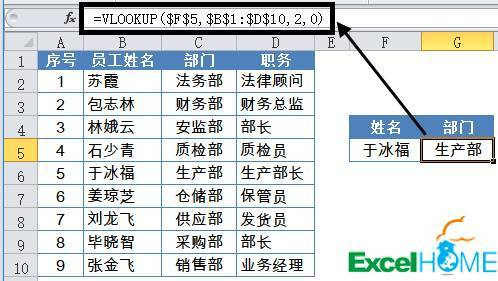1、第4参数一般用0（或FASLE）以精确匹配方式进行查找。
2、第3参数中的列号，不能理解为工作表中实际的列号，而是指定返回值在查找范围中的第几列。
3、如果查找值与数据区域关键字的数据类型不一致，会返回错误值#N/A。
4、查找值必须位于查询区域中的第一列。

8、多条件查找

=LOOKUP(1,0/((B2:B9=F2)*(C2:C9=G2)),A2:A9)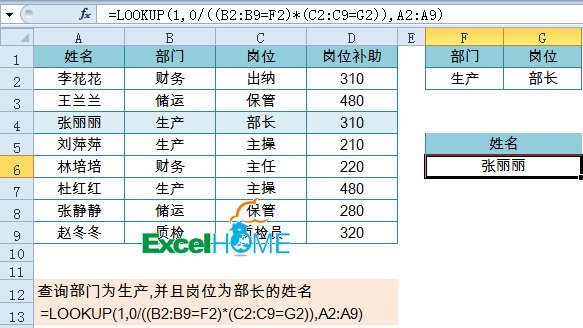LOOKUP函数多条件查询写法为：
=LOOKUP(1,0/((条件区域1=条件1)*(条件区域2=条件2)),查询区域)

9、计算文本算式

=计算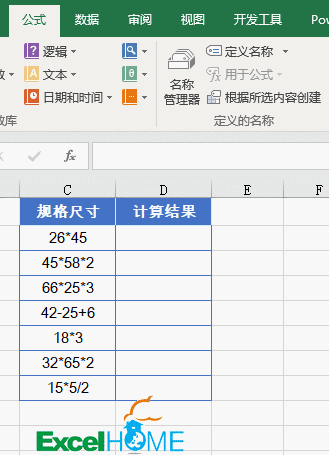10、合并多个单元格内容

=A2&B\$1&B2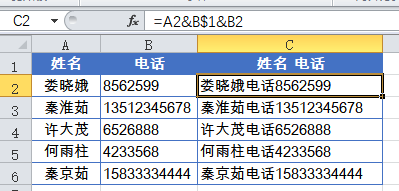11、合并带格式的单元格内容=A2&TEXT(B2," y年m月d日")

12、比较大小写的单词是否相同

=A2=B2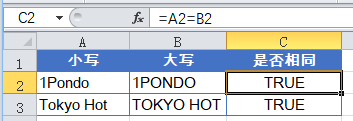=EXACT(A2,B2)
EXACT函数 区分大小写，但忽略格式上的差异。

13、提取混合内容中的姓名

=LEFT(A2,LENB(A2)-LEN(A2))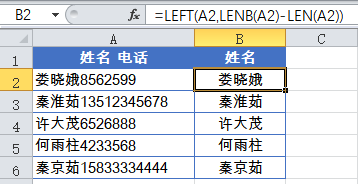LENB函数将每个汉字（双字节字符）的字符数按2计数，LEN函数则对所有的字符都按1计数。因此“LENB(A2)-LEN(A2)”返回的结果就是文本字符串中的汉字个数。
LEFT函数从文本字符串的第一个字符开始，返回指定个数的字符，最终提取出员工姓名。

14、根据身份证号码提取出生年月

=1*TEXT(MID(B2,7,8),"0-00-00")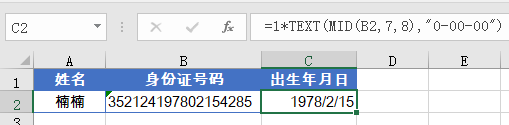"19780215"

"1978-02-15"

15、替换部分电话号码

=SUBSTITUTE(B2,MID(B2,4,4),"**",1)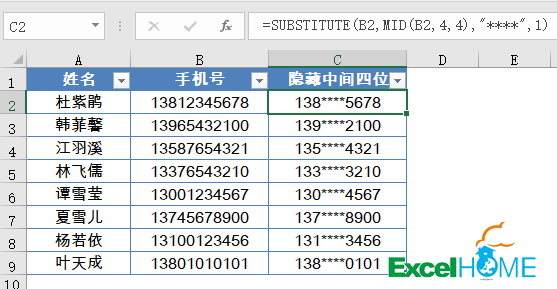SUBSTITUTE函数的用法是：
SUBSTITUTE(要替换的文本,旧文本,新文本,[替换第几个])

16、屏蔽函数公式返回的错误值

IFERROR函数的用法为：
=IFERROR(原公式,出现错误时要返回的内容)

17、四舍五入函数
ROUND函数这个想必大家经常用到吧，就是对数值按指定的位数四舍五入。比如：
=ROUND(8/9,3)

18、取整的间隔小时数

=TEXT(B2-B1,"[h]")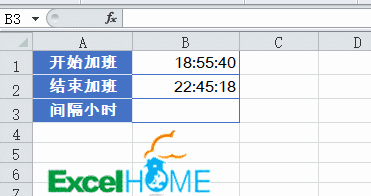19、提取日期时间中的日期值

=INT(A2)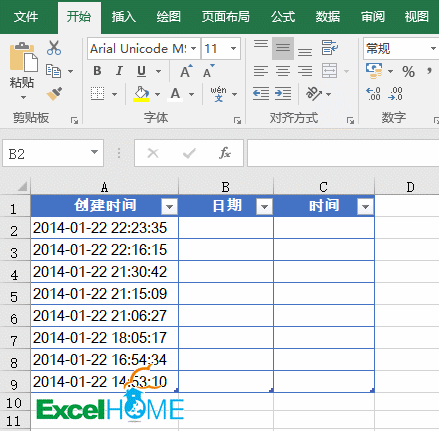20、生成随机数
RANDBETWEEN能够在指定范围内生成一组随机数据，对于广大质检、监理、统计人员来说，这的确是一个伟大的函数。

=RANDBETWEEN(数字下限,数字上限)

=RANDBETWEEN(60,100)### 评论 抢沙发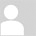• 昵称 (必填)
• 邮箱 (必填)
• 网址